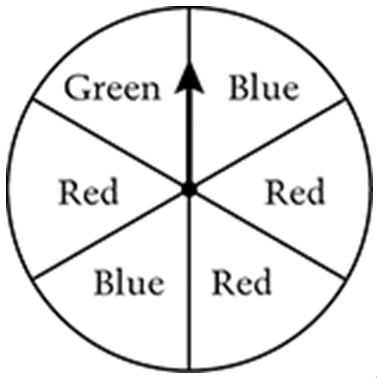# Statistics and Probability / Unequally Likely Outcomes

Activity 14 of 24
In this lesson, students investigate chance processes and generate probability models for events that are not equally likely.

## Planning and Resources

Objectives
Students should recognize a chance event in which the outcomes are not equally likely and find the probabilities of those outcomes.

Vocabulary
equally likely
probability

Standard:

## Lesson Snapshot

#### Understanding

Students recognize the difference between outcomes of a chance event that are equally likely and those that are not.

### What to look for

By the end of this lesson, students should be able to use their experimental results and the theoretical probabilities to explain why the events in a chance process are or are not equally likely.

### Sample Assessment

Are the outcomes in the spinner equally likely? Why or why not?